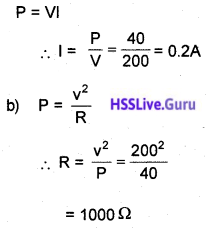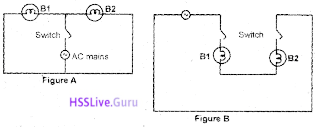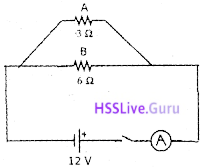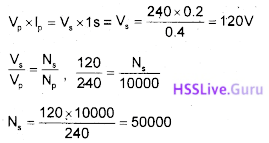# Kerala SSLC Physics Model Question Paper 3 English Medium

Students can Download Kerala SSLC Physics Model Question Paper 3 English Medium Pdf, Kerala SSLC Physics Model Question Papers helps you to revise the complete Kerala State Board New Syllabus and score more marks in your examinations.

## Kerala SSLC Physics Model Question Paper 3 English Medium

General Instructions:

1. The first 15 minutes is the cool off time. You may – use the time to read and plan your answers.
2. Answer the questions only after reading the instructions and questions thoroughly.
3. Questions with marks series 1, 2, 3 and 4 are categorized as sections A, B, C and D respectively.
4. Five questions are given in each section. Answer any four from each section.
5. Answer each question by keeping the time.

Time: 1½ Hours
Total Score: 40 Marks

Section – A

Answer any four questions. Each question carries 1 score. [4 × 1 = 4]

Question 1.
Find the relation and complete the word pair
Transformer: Mutual induction
Inductor : __________.
Self induction

Question 2.
Select the odd one
Cock, ammonia, coal tar, Naphtha
Naphtha

Question 3.
Write down 2 properties of fuse wire.
Low melting point, high resistanceQuestion 4.
Which of the given does not indicate power
I²R, 1/I, IR², V²/R
IR²

Question 5.
What is the relation between anlage of incidence and angle of reflection?
Equal.

Section – B

Answer any 4 questions. Each question carries 2 score. [4 × 2 = 8]

Question 6.
Write down two advantages and two limitations of Hydrogen as a fuel

1. Greater calorific value
2. Easily available

Limitations:

1. Highly inflammable and explosive
2. Difficult to store and transport.Question 7.
The electricity generated at the power stations is transmitted to distant places in high voltage.
a) What is the voltage at which electricity is generated in the power station?
b) What are the problems facing in long distant power transmission?
a) 11 KV
b) Power loss, Voltage drop

Question 8.
Match the following

 A B AC Generator Self Induction Transformer Slip Ring Bio mass Mutual Induction Inductor Green Energy Methane

 A B AC Generator Slip Ring Transformer Mutual Induction Bio mass Green Energy Inductor Self Induction

Question 9.a) What is the intensity of current through the circuit?
b) Calculate the heat developed in the circuit for 20 minutes?
a) I = $$\frac{V}{R}=\frac{10}{30}$$
= 0.3 A

b) H = I²Rt
= 0.3² × 30 × 20 × 60
= 3240 JQuestion 10.
Mention the phenomena responsible for the following
a) Formation of rainbow
b) Tyndal effect
a) Dispersion
b) Scattering

Section – C

Answer any 4 questions. Each question carries 3 score. [4 × 3 = 12]

Question 11.
Observe carefully the following diagram which show the splitting up of a composite light into its constituent coloures.a) Which among the above figure is correct ? Give reason. (2)
b) Write the name of the phenomenon. (1)
a)Light undergoes refraction and the extend of deviation depends on the wavelength. Violet having least wavelength undergoes maximum deviation and red rays having highest wavelength undergoes minimum deviation.
b) Dispersion.

Question 12.
Thickness of insulated copper wire used in the primary turns and secondary turns of a transformer are not equal.
a) In a step up transformer which set of turns (primary/secondary) is made of thicker copper wire? Justify your answer.  (2)
b) Write down the working principle of transformer.   (1)
a) Primary
Reason: In a transformer if there is no power loss, power in the primary coil is equal to the power in the secondary P = Iv, in a step up transformer, in the primary coil, voltage will be low and .current will be high. To carry high current, thick wires are needed.
b) Mutual induction.Question 13.
Wind mill, Hydro electric power, Nuclear reactor and Solar cell are four energy sources.
a) Classify the above four energy sources as green energy and brown energy. (2)
b) What is meant by green energy? (1)
a)

 Green energy Brown energy Wind mill Nuclear reactor Hydro electric power Solar cell

b) Green energy is the energy produced from natural sources which does not cause environmental pollution.

Question 14.
An electric bulb is labelled 40W, 200V.
a) Calculate the current flowing through it?
b) What is the resistance of the filament?
a)Question 15.a) Identify the figure in which bulbs are being connected in series?
b) Select the figure with which the bulbs connected in parallel?
a)b)c) Parallel connection.
Each devices can be controlled by separate switches. Voltage remains same. Effective voltage will be very less.

Section – D

Answer any 4 questions. Each question carries 4 score. [4 × 4 = 16]

Question 16.
White light splits into its constituent colours when passed through a glass prism.
a) Name the process of splitting
b) Give the reasons for this type of splitting
c) Draw the diagram for recombining these colours produced by the prism.
a) Dispersion
b) Each colour differs in their wavelength. Red having greater wavelength deviates least. Violet having less wavelength deviates most. So colour splitted up.
c)Question 17.
Analyse the circuit and answer the following questions.1) What is the current through A?
2) What is the current through B?
3) What will be the ammetdr reading?
4) To decrease the q reading in the ammeter, how should the resistors be connected?
1. Current through A = I1 = $$\frac{v}{R}=\frac{12}{3}$$ = 4A
2. Current through B = I2 = $$\frac{v}{R}=\frac{12}{2}$$ = 2A
3. Ammeter reading = I1 + I2 = 4 + 2 = 6A
4. Resistors should be connected in parallel. Then effective resistance = 3 + 6 = 9W
I = $$\frac{12}{9}$$ = 1.33 A

Question 18.
There are 10000 turns in a transformer. Voltage in the primary is 240 V and current is 0.2 A. The transformer is wound so as to get a current 0.4 A in the secondary.
a) What type of transformer is this?
b) What is the secondary voltage. Also find the number of turns in the secondary.
c) What is the maximum output power that received from this transformer?
a) Step up transformer
b)c) P = VI = 240 × 0.2
= 48 w

Question 19.
When an object is placed infront of a spherical mirror at a distance 30 cm, the magnification is -1.
a) Write down the properties of the image?
b) What kind of mirror is this?
c) If the object is placed at 10 cm. What changes occur to the properties of the image?
d) Illustrate the conclusions.